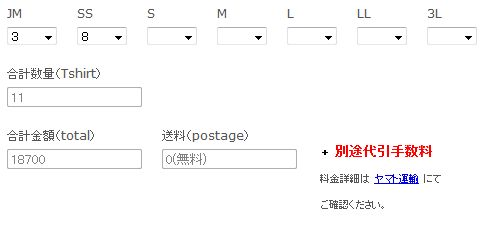# About the re-calculated value

•masaminn
Asked on June 17, 2012 at 10:31 PM

Hello, I am studying JotForm, you [ of JotForm ].
Would you lend me wisdom about the following?

The numerical value inputted by form was re-calculated and it is considered that the value would like to transmit to the form of an automatic reply.

In order to re-calculate the value inputted by JotForm, the script was added to the source code.
The following source codes are taken out from JotForm.

----------------------------------------------------

<li class="form-line form-line-column" id="id_39">
<label class="form-label-top" id="label_39" for="input_39"> total </label>
<div id="cid_39" class="form-input-wide">
<input type="text" class="form-textbox" id="input_39" name="q39_total" size="20" />
</div>
</li>

----------------------------------------------------

The value re-calculated with the following scripts is reput into this.
(Sauce is extracted)

----------------------------------------------------
<script type="text/javascript">
// *****************************
var SizeJM = 'input_32';  //  Tshirt JM
var SizeTotal = 'input_31';  //  Tshirt Total
var PayOutput = 'input_39';  //  Tshirt Pay
var ShpOutput = 'input_40';  //  Tshirt Pay
var ShpFree = '4';  //  Tshirt Pay
var CountA = '1';
var PriceA = '2300';
var CountB = '2';
var PriceB = '2100';
var CountC = '3';
var PriceC = '1800';
var CountD = '10';
var PriceD = '1700';
var CountMAX = '29';
var PriceMAX = '1600';
var BuffPrice = '0';

\$(SizeTotal).disable(); // disable the output field
\$(PayOutput).disable(); // disable the output field
\$(ShpOutput).disable(); // disable the output field

function CheckSize ()
{
//count
\$(SizeTotal).value = Number(\$F(SizeJM))

//ShpFree chk
if (Number(\$F(SizeTotal))  >= ShpFree)
\$(ShpOutput).value = '0(free)';

else \$(ShpOutput).value = '500';

//pay
if (Number(\$F(SizeTotal))  == CountA)
\$(PayOutput).value = PriceA;

else if (Number(\$F(SizeTotal))  == CountB)
\$(PayOutput).value = PriceB * \$(SizeTotal).value;

else if ((Number(\$F(SizeTotal))  >= CountC) & (Number(\$F(SizeTotal))  < CountD))
\$(PayOutput).value = PriceC * \$(SizeTotal).value;

else if ((Number(\$F(SizeTotal))  >= CountD) & (Number(\$F(SizeTotal))  <= CountMAX))
\$(PayOutput).value = PriceD * \$(SizeTotal).value;

else \$(PayOutput).value = PriceMAX * \$(SizeTotal).value;

//event
Event.observe('cid_32', 'change', function(event)
{
CheckSize() ;
});

----------------------------------------------------

In a display top, the form display shown by \$(PayOutput).value is changed as assumption in real time.
However, a value is not reflected in the automatic reply mail sent at the time of form transmission.
What is the method considered since a value is reflected?
Thank you for reading my awkward English•NeilVicente
Answered on June 18, 2012 at 04:13 AM

To be honest, we usually advise against drastic customization of form codes. We cannot guarantee that a highly-customized form will work as intended.

However, we might be able to give some insight if you can share to us the URL of the page where you have inserted the customized form codes (with your own calculation script).